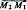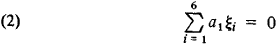# Line Geometry

## Line Geometry

a branch of geometry in which lines are considered as the elements of space. A line in space is determined by four constants—the coefficients a, b, p, and q in the equations x = az + p and y = bz + q. Consequently, the quantities a, b, p, and q may be considered as the coordinates of the line. If these coordinates are functions of one, two, or three parameters, then the corresponding sets of lines form, respectively, ruled surfaces and congruences and complexes of lines. These geometric entities are the objects studied in line geometry. A hyper-boloid of one sheet is an example of a ruled surface, the set of common tangents to two surfaces is an example of a congruence, and the set of tangents to a surface is as an example of a complex of lines.

In order to study ruled surfaces, congruences, and complexes of lines from a unified point of view, we use in line geometry so-called linear homogeneous coordinates of a line. Specifically, given two points M1 (x1, y1, z1) and M2(x2, y2, z2), we call six numbers proportional (or equal) to the numbers

ξ1 = x1 - x2, ξ2 = y1 - y2, ξ3 = z1 - z2, ξ4 = y1z2 - y2z1, ξ5 = x2z1 - x1z2
ξ6 = x1y2 - x2y1

linear homogeneous coordinates of the line that passes through these points.

The numbers ξ1, ξ2, and ξ3 are the components of the vectorand ξ4, ξ5, and ξ6 are the components of the moment of this vector with respect to the origin. It can be easily verified that the numbers ξi satisfy the relation

(1) ξ1ξ4 + ξ2ξ5 + ξ3ξ6 = 0

Thus, to every line there correspond six numbers ξi that are determined up to a multiplicative constant and satisfy relation (1), and, conversely, numbers ξi (not all equal to zero) that are related by condition (1) uniquely define a line (as its coordinates in the sense indicated above).

One homogeneous linear equationdefines a linear complex—a set of lines that fill space in such a way that through every point in space there passes a plane pencil of lines. Thus, to every point (“pole”) in space is associated a plane (“polar plane”) that contains all the lines of the complex passing through that point. This correspondence is called a null system; it is analogous to the correspondence between the poles and polar planes of a quadratic surface. If the polar planes of all points in space pass through one line (the axis), then the complex consists of all lines that intersect the axis; such a complex is called a special linear complex. In this case, the coefficients of equation (2) satisfy the condition a1a4 + a2a5 + a3a6 = 0.

A system of two homogeneous linear equations of the form (2) defines a linear congruence—the set of lines that intersect two given lines (which may be imaginary). Three homogeneous linear equations define a ruled surface, which in this case is either a hyperboloid of one sheet or a hyperbolic paraboloid.

Linear homogeneous coordinates of a line were introduced by J. Plücker in 1846. He also studied in detail the theory of linear complexes. Line geometry subsequently was developed in the works of F. Klein and the Russian mathematician A. P. Kotel’nikov. The differential geometry of congruences initiated by E. Kummer in 1860 was developed extensively in the works of the Italian mathematicians L. Bianchi and G. Sannia and the French mathematician A. Ribaucour. The theory of ruled surfaces and congruences was developed by the Soviet mathematician D. N. Zeiliger on the basis of the “spiral” calculus devised in 1895 by Kotel’nikov. The projective theory of congruences was constructed in 1927 by the Soviet mathematician S. P. Fini-kov.

### REFERENCES

Zeiliger, D. N. Kompleksnaia lineichataia geometriia: Poverkhnosti i kongruentsii. Leningrad-Moscow, 1934.
Finikov, S. P. Teoriia poverkhnostei. Moscow-Leningrad, 1934.
Finikov, S. P. Proektivno-differentsial’naia geometriia. Moscow-Leningrad, 1937.
Finikov, S. P. Teoriia kongruentsii. Moscow-Leningrad, 1950.
Kagan, V. F. Osnovy teorii poverkhnostei v tenzornom izlozhenii, parts 1–2. Moscow-Leningrad, 1947–48.
Klein, F. Vysshaia geometriia. Moscow-Leningrad, 1939. (Translated from German.)
Zindler, K. Liniengeometrie, vols. 1–2. Leipzig, 1902–06.

E. G. POZNIAK

Mentioned in ?
References in periodicals archive ?
In this context, traditionally the position of the overhead line geometry in the railway installations is determined manually by the crew in charge of the assembly and maintenance.
The inspection showed the storage tank line geometry consisted of one 45[degrees] (1.5D) bend about 29 feet from the entry point, followed by a 141-foot straight section, an overbend ending with a downward pitch of 18[degrees], and a 100-foot straight section, where the inspection route ended with a stinger running through the center of the pipe.
Although simulation and test correlation had been conducted for available prototype units, FEA modeling error of piping resonant frequency predictions exist due to factors such as simulation assumptions and approximations, and uncertain line geometry when no fixtures were available to build prototype test units, .
Furthermore, there Is no provision in ceramic and ferrite based CMFs for electrostatic discharge (ESD) protection, something which is vital for smartphones using fine line geometry chipsets which are highly susceptible to ESD damage.
The dispersive effective permittivity, [[epsilon].sub.eff] (f), and permeability [[mu].sub.eff](f), of the microstrip line geometry for demagnetized state, are computed from the following equations as [15, 18]
Two installations in Poland and Germany have shown how line geometry can be adapted to fit available space, Cincinnati Extrusion is using its KryoSys system for forced cooling of pipes in a line for Polish producer KNG Awans, while Battenfeld Extrusionstechnik customised a coating line to increase production of plastic-jacketed steel pipe at Salzgitter Mannesmann Line Pipe in Germany.
Coplanar waveguide (CPW) is a transmission line geometry that includes a central current-carrying trace on top of a dielectric substrate, with side grounds extending beyond a symmetric gap to either side of trace.
Finally, different changes in the exhaust line geometry have been investigated by simulation, moving the under-floor SCR to a close coupled position with volume variations.
Controlled Impedance Line Designer for quickly and accurately optimizing stack-up and line geometry for multi-gigabit-per-second chip-to-chip links.
A subgeometry of [GAMMA] is a point line geometry [GAMMA]' = (P', L'), such that P' [subset or equal to] P, L' [subset or equal to] L, and the graph of [GAMMA]' is a subgraph of [GAMMA]; we denote P([GAMMA]') = P' and L([GAMMA]') = L'.
Session 15 on "Joining of plastic parts" will include the following presentations: "Laser transmission welding of parts with a complex weld line geometry," R.

Site: Follow: Share:
Open / Close